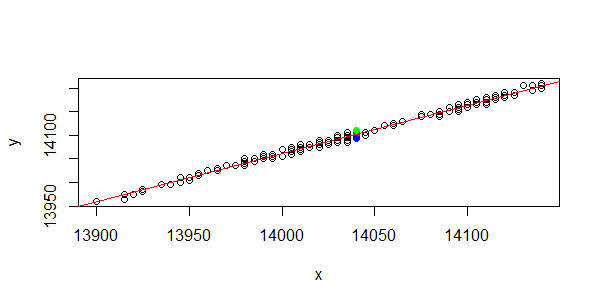• Posts tagged "F检验"

# Blog Archives

## 用R语言解读统计检验-F检验

R的极客理想系列文章，涵盖了R的思想，使用，工具，创新等的一系列要点，以我个人的学习和体验去诠释R的强大。

R语言作为统计学一门语言，一直在小众领域闪耀着光芒。直到大数据的爆发，R语言变成了一门炙手可热的数据分析的利器。随着越来越多的工程背景的人的加入，R语言的社区在迅速扩大成长。现在已不仅仅是统计领域，教育，银行，电商，互联网….都在使用R语言。

• 张丹(Conan), 程序员/Quant: Java,R,Nodejs
• blog: http://blog.fens.me
• email: bsspirit@gmail.com

http://blog.fens.me/r-test-f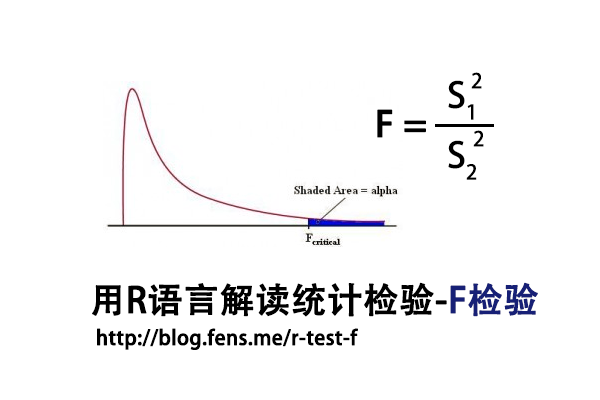1. F检验介绍
2. 数据集
3. F检验实现

## 1. F检验介绍

F检验法(F-test)，初期叫方差比率检验(Variance Ratio)，又叫联合假设检验(Joint Hypotheses Test)，是英国统计学家Fisher提出的，主要通过比较两组数据的方差，以确定他们的密度是否有显著性差异。至于两组数据之间是否存在系统误差，则在进行F检验并确定它们的密度没有显著性差异之后，再进行T检验

F检验，是一种在零假设(H0)之下，统计值服从F-分布的检验。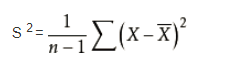F统计量计算公式：

• F:统计量，根据自由度查表，当F值小于查表值时没有显著差异，当F值大于等于查表值时有显著差异
• S1:样本1的标准差
• S2:样本2的标准差
• 分子自由度: df=分子的数量-1
• 分母自由度: df=分母的数量-1

T检验和F检验对比
T检验用来检测数据的准确度(系统误差)，F检验用来检测数据的精密度(偶然误差)。在定量分析过程中，常遇到两种情况：一种是样本测量的平均值与真值不一致；另一种是两组测量的平均值不一致。

T检验是检查两组均值的差异，而F检验是检查多组均值之间的差异。

## 2. 数据集

F检验，对于数据有比较严格的要求，所以我们需要先找到一个合适的数据集，作为测试数据集。我发现了R语言自带的一个数据集ToothGrowth，是很好的测试数据集，本文接下来的内容，将以这个数据集进行测试，来介绍F检验。

• Win10 64bit
• R: 3.4.2 x86_64-w64-mingw32/x64 b4bit

• len列，为牙齿长度
• supp列，为注射方法
• dose列，为注射剂量

``````
> head(ToothGrowth,10)
len supp dose
1   4.2   VC  0.5
2  11.5   VC  0.5
3   7.3   VC  0.5
4   5.8   VC  0.5
5   6.4   VC  0.5
6  10.0   VC  0.5
7  11.2   VC  0.5
8  11.2   VC  0.5
9   5.2   VC  0.5
10  7.0   VC  0.5
``````

F检验对于数据的正态性非常敏感，我们需要先对选定数据集进行进行正态分布检验。使用Shapiro-Will作为正态分布检验的方法，原假设H0:样本符合正态分布。

``````
# 按不同的处理方法，进行分组
> len_VC<-ToothGrowth\$len[which(ToothGrowth\$supp=='VC')]
> len_OJ<-ToothGrowth\$len[which(ToothGrowth\$supp=='OJ')]

# 正态分布检验
> shapiro.test(len_VC)

Shapiro-Wilk normality test

data:  len_VC
W = 0.96567, p-value = 0.4284

# 正态分布检验
> shapiro.test(len_OJ)

Shapiro-Wilk normality test

data:  len_OJ
W = 0.91784, p-value = 0.02359
``````

``````
> coplot(len ~ dose | supp, data = ToothGrowth, panel = panel.smooth,
xlab = "ToothGrowth data: length vs dose, given type of supplement")
``````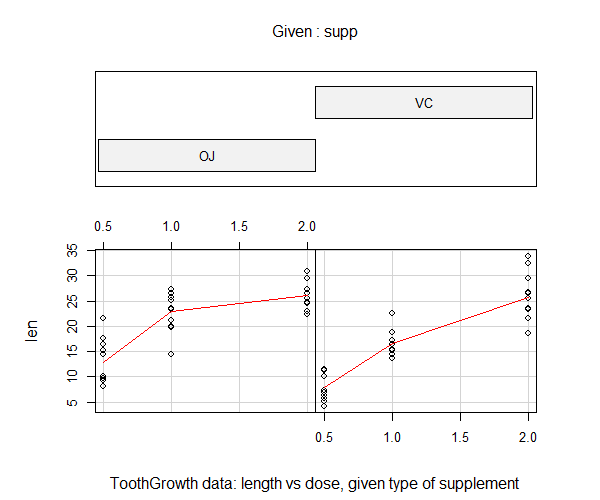## 3. F检验实现

3.1 随机数进行F检验

``````
# 生成随机数
> set.seed(1)
> x <- rnorm(50, mean = 0, sd = 2)
> y <- rnorm(30, mean = 1, sd = 1)

# 进行F检验
> var.test(x, y)

F test to compare two variances

data:  x and y
F = 2.6522, num df = 49, denom df = 29, p-value
= 0.006232
alternative hypothesis: true ratio of variances is not equal to 1
95 percent confidence interval:
1.332510 4.989832
sample estimates:
ratio of variances
2.652168
``````

• H0:原假设2组样本的方差，无显著差异
• F统计量：2.6522
• num df，分子自由度，50-1=49
• denom df，分每自由度，30-1=29
• p-value值：0.006232
• 95 percent confidence interval：95%的置信区间
• ratio of variances：方差比率2.652168

3.2 ToothGrowth进行F检验

``````
> var.test(len_VC,len_OJ)

F test to compare two variances

data:  len_VC and len_OJ
F = 1.5659, num df = 29, denom df = 29, p-value
= 0.2331
alternative hypothesis: true ratio of variances is not equal to 1
95 percent confidence interval:
0.745331 3.290028
sample estimates:
ratio of variances
1.565937
``````

``````
# 手动计算T值
> Xn<-length(len_VC)
> Yn<-length(len_OJ)
> Xm<-mean(len_VC)
> Ym<-mean(len_OJ)

# 计算两组样本的偏方差
> fx<-sum((len_VC-Xm)^2)/(Xn-1)
> fy<-sum((len_OJ-Ym)^2)/(Yn-1)

# 计算F值
> fx/fy
 1.565937

# 手动计算P值，双边检验
> p_value<-pf(f_stat,Yn-1,Xn-1)
> p_value<-2*min(p_value, 1 - p_value);p_value
 0.2331433
``````

http://blog.fens.me/r-test-f## R语言解读一元线性回归模型

R的极客理想系列文章，涵盖了R的思想，使用，工具，创新等的一系列要点，以我个人的学习和体验去诠释R的强大。

R语言作为统计学一门语言，一直在小众领域闪耀着光芒。直到大数据的爆发，R语言变成了一门炙手可热的数据分析的利器。随着越来越多的工程背景的人的加入，R语言的社区在迅速扩大成长。现在已不仅仅是统计领域，教育，银行，电商，互联网….都在使用R语言。

• 张丹(Conan), 程序员R,Nodejs,Java
• weibo：@Conan_Z
• blog: http://blog.fens.me
• email: bsspirit@gmail.com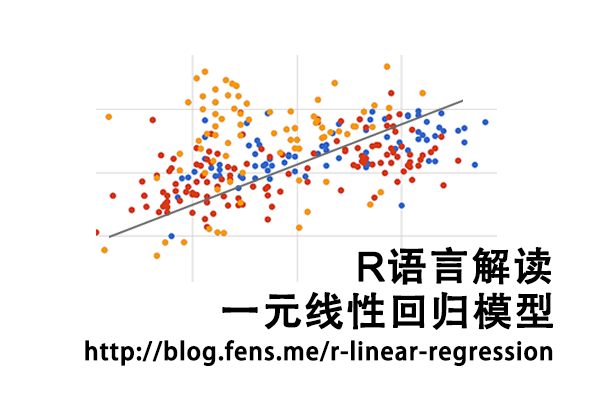1. 一元线性回归介绍
2. 数据集和数学模型
3. 回归参数估计
4. 回归方程的显著性检验
5. 残差分析和异常点检测
6. 模型预测

## 1. 一元线性回归介绍

1. 确定因变量Y 与 自变量X1, X2, …, Xn 之间的定量关系表达式，即回归方程。
2. 对回归方程的置信度检查。
3. 判断自变量Xn(n=1,2,…,m)对因变量的影响。
4. 利用回归方程进行预测。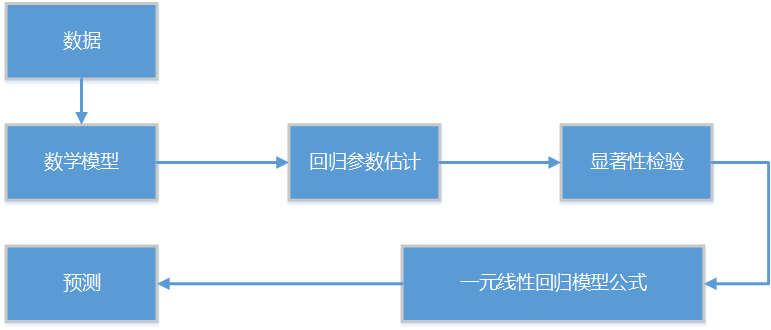## 2. 数据集和数学模型

``````
zn1.Close zn2.Close
2016-03-01 09:01:00     14075     14145
2016-03-01 09:02:00     14095     14160
2016-03-01 09:03:00     14095     14160
2016-03-01 09:04:00     14095     14165
2016-03-01 09:05:00     14120     14190
2016-03-01 09:06:00     14115     14180
2016-03-01 09:07:00     14110     14170
2016-03-01 09:08:00     14110     14175
2016-03-01 09:09:00     14105     14170
2016-03-01 09:10:00     14105     14170
2016-03-01 09:11:00     14120     14180
2016-03-01 09:12:00     14105     14170
2016-03-01 09:13:00     14105     14170
2016-03-01 09:14:00     14110     14175
2016-03-01 09:15:00     14105     14175
2016-03-01 09:16:00     14120     14185
2016-03-01 09:17:00     14125     14190
2016-03-01 09:18:00     14115     14185
2016-03-01 09:19:00     14135     14195
2016-03-01 09:20:00     14125     14190
2016-03-01 09:21:00     14135     14205
2016-03-01 09:22:00     14140     14210
2016-03-01 09:23:00     14140     14200
2016-03-01 09:24:00     14135     14205
2016-03-01 09:25:00     14140     14205
2016-03-01 09:26:00     14135     14205
2016-03-01 09:27:00     14130     14205
``````

``````
# 数据集已存在df变量中
> head(df)
zn1.Close zn2.Close
2016-03-01 09:01:00     14075     14145
2016-03-01 09:02:00     14095     14160
2016-03-01 09:03:00     14095     14160
2016-03-01 09:04:00     14095     14165
2016-03-01 09:05:00     14120     14190
2016-03-01 09:06:00     14115     14180

# 分别给x,y赋值
> x<-as.numeric(df[,1])
> y<-as.numeric(df[,2])

# 画图
> plot(y~x+1)
``````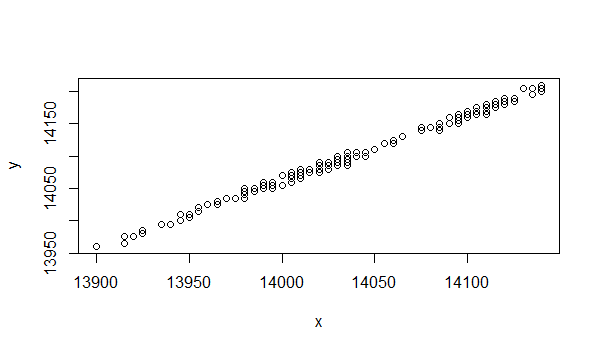``Y = a + b * X + c``
• Y，为因变量
• X，为自变量
• a，为截距
• b，为自变量系数
• a+b*X, 表示Y随X的变化而线性变化的部分
• c, 为残差或随机误差，是其他一切不确定因素影响的总和，其值不可观测。假定c是符合均值为0方差为σ^2的正态分布 ，记作c~N(0,σ^2)

``````
Yi = a + b * X + ci，     i= 1,2,...n

``````

## 3. 回归参数估计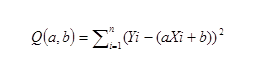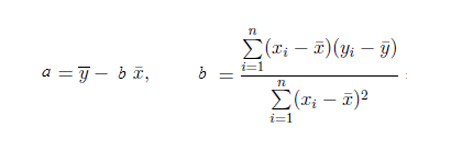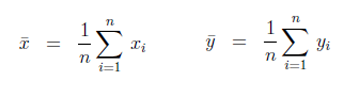``````
# 建立线性回归模型
> lm.ab<-lm(y ~ 1+x)

# 打印参数估计的结果
> lm.ab

Call:
lm(formula = y ~ 1 + x)

Coefficients:
(Intercept)            x
-349.493        1.029
``````

``````
# x均值
> Xm<-mean(x);Xm
 14034.82

# y均值
> Ym<-mean(y);Ym
 14096.76

# 计算回归系数
> b <- sum((x-Xm)*(y-Ym)) / sum((x-Xm)^2) ;b
 1.029315

# 计算回归常数
> a <- Ym - b * Xm;a
 -349.493
``````

``Y= a + b * X = -349.493 + 1.029315 * X ``

``````
> plot(y~x+1)
> abline(lm.ab)
``````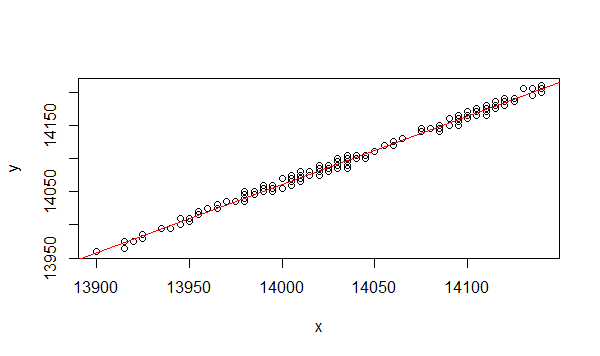## 4. 回归方程的显著性检验

• T检验法：T检验是检验模型某个自变量Xi对于Y的显著性，通常用P-value判断显著性，小于0.01更小时说明这个自变量Xi与Y相关关系显著。
• F检验法：F检验用于对所有的自变量X在整体上看对于Y的线性显著性，也是用P-value判断显著性，小于0.01更小时说明整体上自变量与Y相关关系显著。
• R^2(R平方)相关系统检验法：用来判断回归方程的拟合程度，R^2的取值在0，1之间，越接近1说明拟合程度越好。

``````
> summary(lm.ab)      # 计算结果

Call:
lm(formula = y ~ 1 + x)

Residuals:
Min       1Q   Median       3Q      Max
-11.9385  -2.2317  -0.1797   3.3546  10.2766

Coefficients:
Estimate Std. Error t value Pr(>|t|)
(Intercept) -3.495e+02  7.173e+01  -4.872 2.09e-06 ***
x            1.029e+00  5.111e-03 201.390  < 2e-16 ***
---
Signif. codes:  0 ‘***’ 0.001 ‘**’ 0.01 ‘*’ 0.05 ‘.’ 0.1 ‘ ’ 1

Residual standard error: 4.232 on 223 degrees of freedom
Multiple R-squared:  0.9945,	Adjusted R-squared:  0.9945
F-statistic: 4.056e+04 on 1 and 223 DF,  p-value: < 2.2e-16

``````

• Call，列出了回归模型的公式。
• Residuals，列出了残差的最小值点，1/4分位点，中位数点，3/4分位点，最大值点。
• Coefficients，表示参数估计的计算结果。
• Estimate，为参数估计列。Intercept行表示常数参数a的估计值 ，x行表示自变量x的参数b的估计值。
• Std. Error，为参数的标准差，sd(a), sd(b)
• t value，为t值，为T检验的值
• Pr(>|t|) ，表示P-value值，用于T检验判定，匹配显著性标记
• 显著性标记，***为非常显著，**为高度显著, **为显著，·为不太显著，没有记号为不显著。
• Residual standard error，表示残差的标准差，自由度为n-2。
• Multiple R-squared，为相关系数R^2的检验，越接近1则越显著。
• Adjusted R-squared，为相关系数的修正系数，解决多元回归自变量越多，判定系数R^2越大的问题。
• F-statistic，表示F统计量，自由度为(1,n-2)，p-value:用于F检验判定，匹配显著性标记。

``Y = -349.493 + 1.029315 * X ``

## 5. 残差分析和异常点检测

``````
# 残差
> y.res<-residuals(lm.ab)

# 打印前6条数据
> head(y.res)
1         2         3         4         5         6
6.8888680 1.3025744 1.3025744 6.3025744 5.5697074 0.7162808

# 正态分布检验
> shapiro.test(y.res)

Shapiro-Wilk normality test

data:  y.res
W = 0.98987, p-value = 0.1164

# 画出残差散点图
> plot(y.res)
``````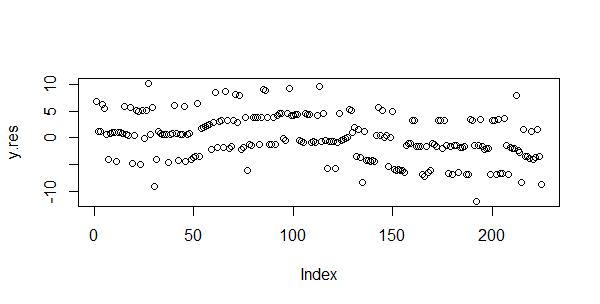``````
# 画图，回车展示下一张
> plot(lm.ab)
Hit  to see next plot:   # 残差拟合图
Hit  to see next plot:   # 残差QQ图
Hit  to see next plot:   # 标准化的残差对拟合值
Hit  to see next plot:   # 标准化残差对杠杆值
``````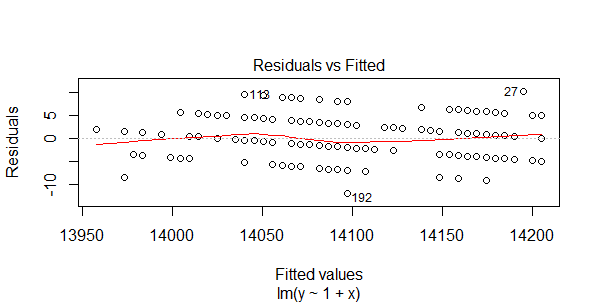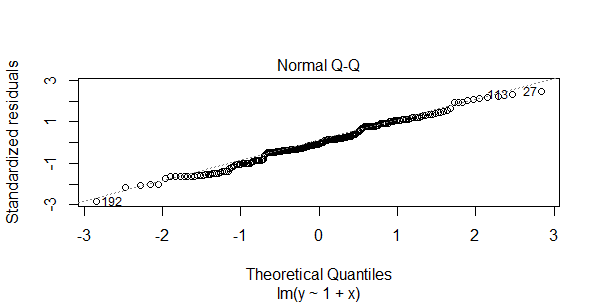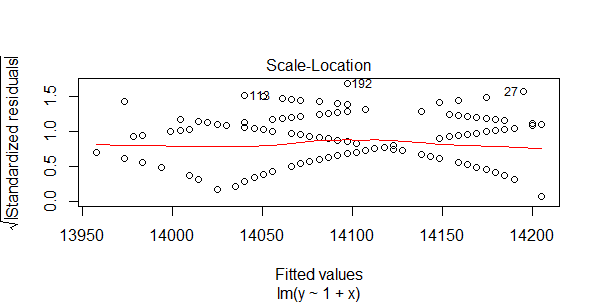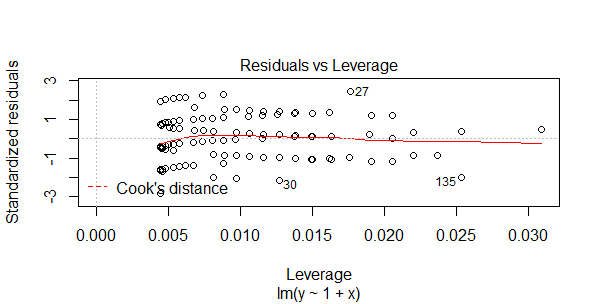``````
> par(mfrow=c(2,2))
> plot(lm.ab)
``````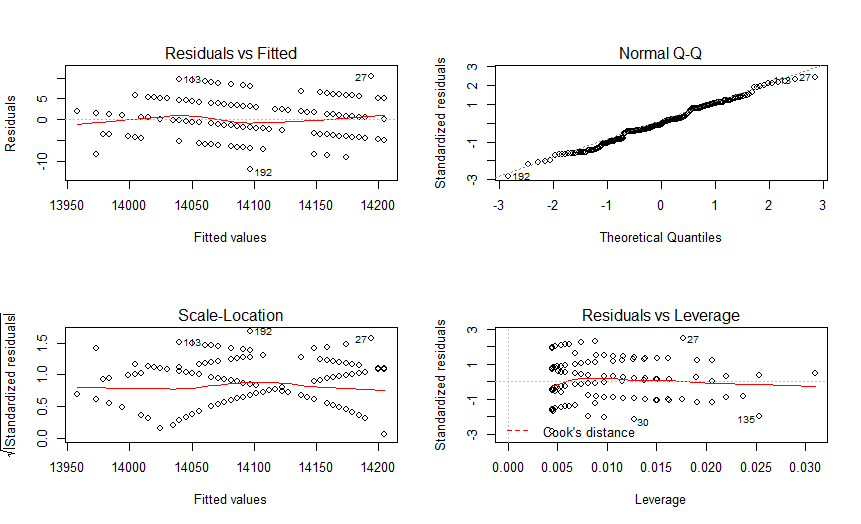``````
# 查看27和192
> df[c(27,192),]
zn1.Close zn2.Close
2016-03-01 09:27:00     14130     14205
2016-03-01 14:27:00     14035     14085

# 新建数据集，去掉27和192
> df2<-df[-c(27,192),]
``````

``````
> x2<-as.numeric(df2[,1])
> y2<-as.numeric(df2[,2])
> lm.ab2<-lm(y2 ~ 1+x2)
> summary(lm.ab2)

Call:
lm(formula = y2 ~ 1 + x2)

Residuals:
Min      1Q  Median      3Q     Max
-9.0356 -2.1542 -0.2727  3.3336  9.5879

Coefficients:
Estimate Std. Error t value Pr(>|t|)
(Intercept) -3.293e+02  7.024e+01  -4.688 4.83e-06 ***
x2           1.028e+00  5.004e-03 205.391  < 2e-16 ***
---
Signif. codes:
0 ‘***’ 0.001 ‘**’ 0.01 ‘*’ 0.05 ‘.’ 0.1 ‘ ’ 1

Residual standard error: 4.117 on 221 degrees of freedom
Multiple R-squared:  0.9948,	Adjusted R-squared:  0.9948
F-statistic: 4.219e+04 on 1 and 221 DF,  p-value: < 2.2e-16
``````

## 6. 模型预测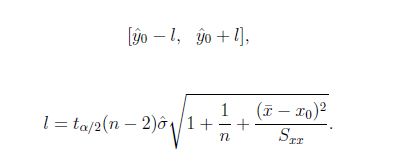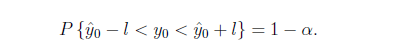``````
> new<-data.frame(x=14040)
> lm.pred<-predict(lm.sol,new,interval="prediction",level=0.95)

# 预测结果
> lm.pred
fit      lwr      upr
1 14102.09 14093.73 14110.44
``````

``````
> plot(y~x+1)
> abline(lm.ab,col='red')
> points(rep(newX\$x,3),y=lm.pred,pch=19,col=c('red','blue','green'))
``````